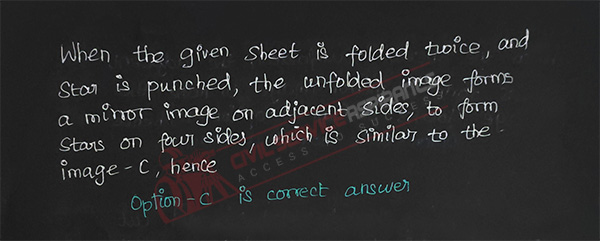# Paper Folding UPSC Questions

## Paper Folding MCQ Questions

7.

In a figure a transparent sheet is given. Find out the pattern formed when the transparent sheet is folded . (Dotted line represent the folding of paper)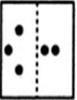A.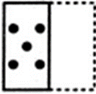B.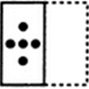C.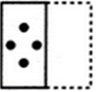D.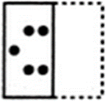B.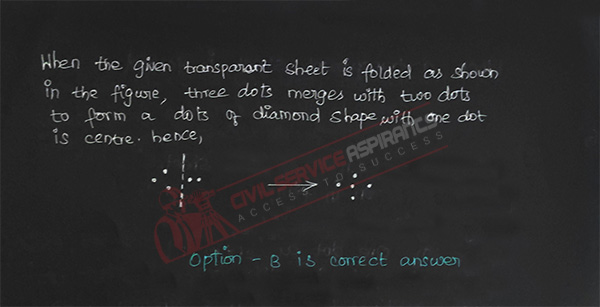8.

In a figure a transparent sheet is given. Find out the pattern formed when the transparent sheet is folded . (Dotted line represent the folding of paper)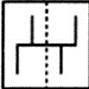A.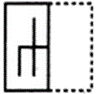B.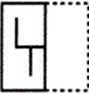C.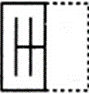D.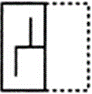C.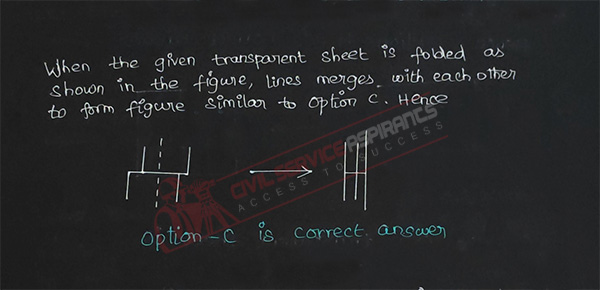9.

In a figure a transparent sheet is given. Find out the pattern formed when the transparent sheet is folded . (Dotted line represent the folding of paper)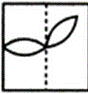A.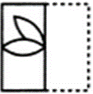B.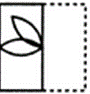C.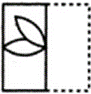D.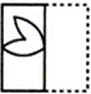B.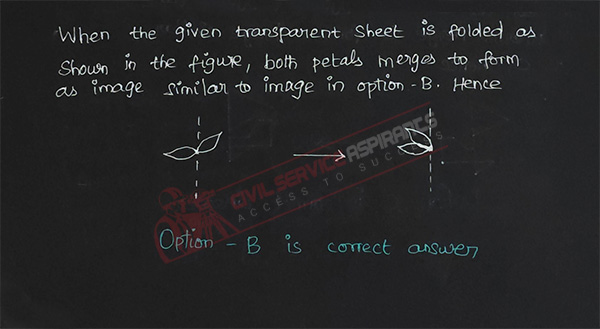10.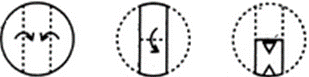A.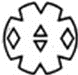B.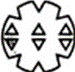C.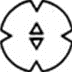D.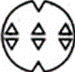D.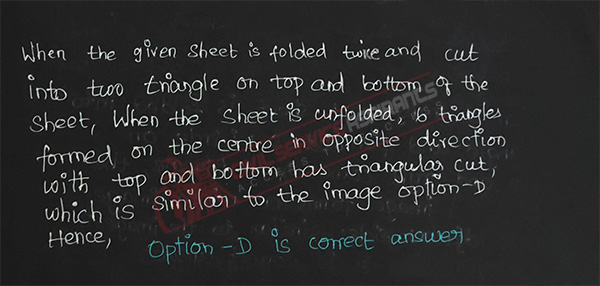11.

Find the image which would mostly matches with the unfolded form given below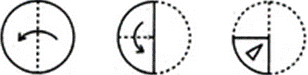A.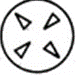B.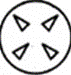C.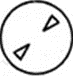D.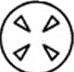D.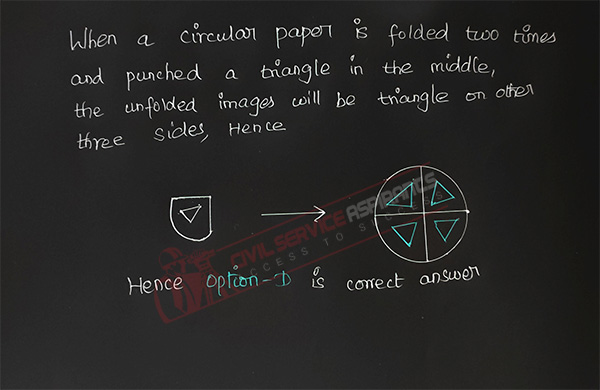12.

Find the image which would mostly matches with the unfolded form given below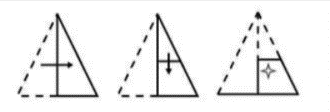A.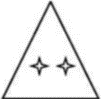B.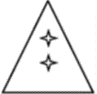C.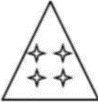D.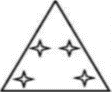C.